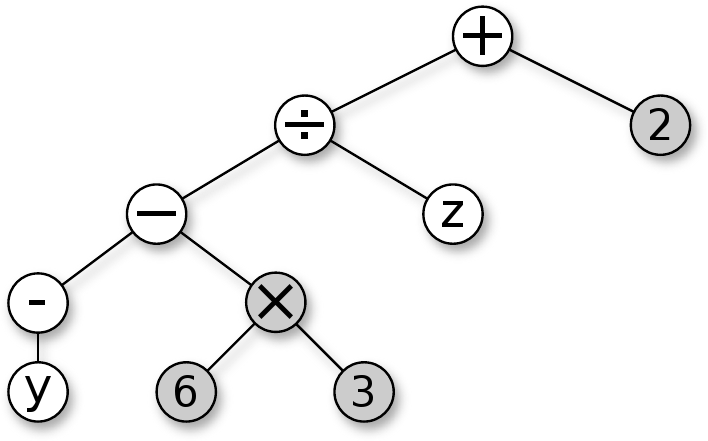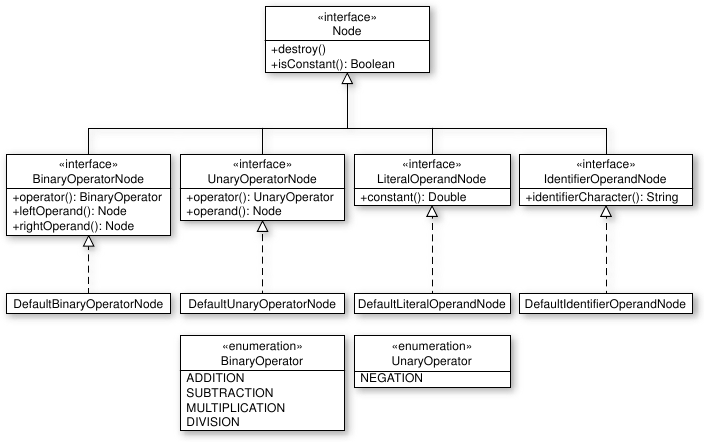# 3.2 A syntax tree representing an arithmetic expression in C

Arithmetic expressions are traditionally written using infix notation, in which operators are written between operands, and parentheses and precedence rules are used to resolve ambiguity (5 + 2/3 is a simple example). As most popular programming languages use infix notation, compiler front-ends must parse such expressions (typically using so-called operator-precedence parsers). The result of such a process is often an unambiguous syntax tree that can be used for further processing. The nodes of a syntax tree for arithmetic expressions are the focus of this example.

Four different classes of nodes are used here:

• Binary operator nodes. Such nodes hold two operands and a binary operator, such as addition, subtraction, multiplication or division. The operands may be arbitrary nodes. A binary operator node is considered to be constant if both its operands are constant.

• Unary operator nodes. Such nodes hold only one operand and a unary operator, such as negation or a trigonometric operator. The operand may be an arbitrary node. A unary operator is considered to be constant if its sole operand is constant.

• Literal operand nodes. Such nodes hold a literal, constant value. A literal operand node is considered to be constant at all times, per definition.

• Identifier operand nodes. Such nodes hold the name of an identifier, which may be either a variable or a constant. An identifier operand node is considered to be constant if, and only if, the identifier it refers to is a constant.

Figure 3.1 shows one possible syntax tree that may result after parsing a sample arithmetic expression. Constant nodes are shown in gray. (Being able to differentiate between constant and variable nodes allows for a common optimization known as constant folding, which entails replacing fully constant subtrees with literal operand nodes representing their values. The expression of Figure 3.1 would be written ((-y - 18) / z) + 2 after folding constants.)1Figure 3.1: Syntax tree corresponding to the expression ((-y - 6 * 3) / z) + 2

Nodes are naturally modeled as objects, instantiated from one of four classes, which in turn serve as the default implementations of four interfaces, corresponding to the classes of nodes listed above. This is depicted in Figure 3.2, using Unified Modeling Language (UML) syntax. For instance, objects modeling binary operator nodes are instances of classes implementing the BinaryOperatorNode interface. All objects modeling nodes also implement the Node interface, as all four domain-specific node interfaces extend this interface. All nodes may be explicitly destroyed, and all nodes may be queried as to whether they and their children are fully constant. The DefaultBinaryOperatorNode class is the sole implementation of the BinaryOperatorNode interface.Figure 3.2: Diagram of an interface hierarchy for arithmetic nodes

This example is interesting in that it is highly dependent on dynamic dispatch. An instance of DefaultBinaryOperatorNode holds two operands, of which the only known fact is that they implement the Node interface. When Node::IsConstant() is called on an instance of DefaultBinaryOperatorNode, it recursively calls this operation on its two operands, and returns true if, and only if, both return true. It is unaware of the precise implementation used by its operands, and must therefore find the implementation at runtime, bringing late binding into play.

Footnotes

1. This simplistic approach to constant folding will not necessarily work for all arithmetic expressions. The expression x + 4 + 3 may be correctly represented as a binary operator node adding 3 to another binary operator node, which adds x to 4, in other words (x + 4) + 3. Neither binary operator node is considered constant in such a syntax tree, and thus no folding of constants will take place. This problem may be solved by rearranging nodes in such a way that constant nodes (such as 4 and 3) are grouped together, while respecting the rules governing the operators. As addition is an associative operator, (x + 4) + 3 can also be written as x + (4 + 3), which may be represented as a binary operator node adding x to another binary operator node, which adds 4 to 3. The latter node is constant, and may be replaced with the literal operand node 7, yielding the expression x + 7.